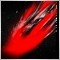# Script for StopOrders On Mouse Function.106

hi

i need to add number of orders = 5 to this script, but unable to do so as price is not considered because it's a drag and drop on mouse script, i do not want to change the script of mouse simplicity, but want to add "number of orders" to the script, meaning multiple pending orders such as (BUYSTOP OR SELLSTOP) will be created of the same price.
kindly help.

```#property show_inputs

//--------------------------------------------------------------------
extern int     Stoploss          = 50,     //
Takeprofit        = 80;     //
extern double  Lot               = 0.01;   //
extern int     Magic             = 0;     //
extern bool    comment           = true;  //display information on screen
//--------------------------------------------------------------------
int start()
{
double SL,TP;
double Price = NormalizeDouble(WindowPriceOnDropped(),Digits);
string txt=StringConcatenate("Stop the script issuing a warrant ",DoubleToStr(Price,Digits)," ñòàðò ",TimeToStr(TimeCurrent(),TIME_DATE|TIME_SECONDS));
RefreshRates();
{
if (Takeprofit!=0) TP = NormalizeDouble(Price + Takeprofit * Point,Digits); else TP=0;
if (Stoploss!=0)   SL = NormalizeDouble(Price - Stoploss   * Point,Digits); else SL=0;
else txt = StringConcatenate(txt,"\nError ",GetLastError()," BUYSTOP ");
}
if(Price<Bid)
{
if (Takeprofit!=0) TP = NormalizeDouble(Price - Takeprofit * Point,Digits); else TP=0;
if (Stoploss!=0)   SL = NormalizeDouble(Price + Stoploss   * Point,Digits); else SL=0;
if (OrderSend(Symbol(),OP_SELLSTOP,Lot,Price,0,SL,TP,"StopOrders",Magic,0,CLR_NONE)==-1)
txt = StringConcatenate(txt,"\nSet SELLSTOP ",DoubleToStr(Price,Digits));
else txt = StringConcatenate(txt,"\nError ",GetLastError()," SELLSTOP ");
}
if (comment) Comment(txt,"\nThe script has finished its work ",TimeToStr(TimeCurrent(),TIME_DATE|TIME_SECONDS));
return(0);
}
//--------------------------------------------------------------------

//--------------------------------------------------------------------

```21915

Yui kmitokawa: i need… kindly help.
Help you with what? You haven't stated a problem, you stated a want. Show us your attempt (using the CODE button) and state the nature of your problem.
No free help 2017.04.21

Or pay someone. Top of every page is the link Freelance.
Hiring to write script - General - MQL5 programming forum 2018.05.1249079

Yui kmitokawa:

hi

i need to add number of orders = 5 to this script, but unable to do so as price is not considered because it's a drag and drop on mouse script, i do not want to change the script of mouse simplicity, but want to add "number of orders" to the script, meaning multiple pending orders such as (BUYSTOP OR SELLSTOP) will be created of the same price.
kindly help.

```#property show_inputs

//--------------------------------------------------------------------
extern int     Stoploss          = 50,     //
Takeprofit        = 80;     //
extern double  Lot               = 0.01;   //
extern int     Magic             = 0;     //
extern bool    comment           = true;  //display information on screen
//--------------------------------------------------------------------
int start()
{

double point = _Point;
if(Digits == 3 || Digits == 5)point = 10.0 * point;

double SL,TP;
double Price = NormalizeDouble(WindowPriceOnDropped(),Digits);
string txt=StringConcatenate("Stop the script issuing a warrant ",DoubleToStr(Price,Digits)," ñòàðò ",TimeToStr(TimeCurrent(),TIME_DATE|TIME_SECONDS));
RefreshRates();
{
if (Takeprofit!=0) TP = NormalizeDouble(Price + Takeprofit * point,Digits); else TP=0;
if (Stoploss!=0)   SL = NormalizeDouble(Price - Stoploss   * point,Digits); else SL=0;
else txt = StringConcatenate(txt,"\nError ",GetLastError()," BUYSTOP ");
}
if(Price<Bid)
{
if (Takeprofit!=0) TP = NormalizeDouble(Price - Takeprofit * point,Digits); else TP=0;
if (Stoploss!=0)   SL = NormalizeDouble(Price + Stoploss   * point,Digits); else SL=0;
if (OrderSend(Symbol(),OP_SELLSTOP,Lot,Price,0,SL,TP,"StopOrders",Magic,0,CLR_NONE)==-1)
txt = StringConcatenate(txt,"\nSet SELLSTOP ",DoubleToStr(Price,Digits));
else txt = StringConcatenate(txt,"\nError ",GetLastError()," SELLSTOP ");
}
if (comment) Comment(txt,"\nThe script has finished its work ",TimeToStr(TimeCurrent(),TIME_DATE|TIME_SECONDS));
return(0);
}
//--------------------------------------------------------------------

//--------------------------------------------------------------------```106

Pavel Shutovskiy:

i tried the point function but it's not working, i even tried modifying normalize function but it's not creating multiple orders.Moderator
23533

`extern int     NumberOfOrders       = 5; //Number of Orders`

and before each OrderSend()

`for(int x=0;x<NumberOfOrders;x++)`

Not tested106

Keith Watford:

and before each OrderSend()

Not tested

yes i did tried that too, thanks Keith, but it's not working.Moderator
23533

Yui kmitokawa:
yes i did tried that too, thanks Keith, but it's not working.

What errors are you getting?

Your original script did work did it?106

Keith Watford:

What errors are you getting?

Your original script did work did it?

there are no errors, but output is not affected.Moderator
23533

Yui kmitokawa:
there are no errors, but output is not affected.106

Keith Watford:

kindly have a look,

```#property show_inputs

//--------------------------------------------------------------------
extern int     Stoploss          = 50,     //
Takeprofit        = 80;     //
extern double  Lot               = 0.01;   //
extern int     NumberOfOrders    = 5;   //Number of Orders
extern int     Magic             = 0;      //
extern bool    comment           = true;   //display information on screen
//--------------------------------------------------------------------
int start()
{
double SL,TP;
double Price = NormalizeDouble(WindowPriceOnDropped(),Digits);
string txt=StringConcatenate("Stop the script issuing a warrant ",DoubleToStr(Price,Digits)," ñòàðò ",TimeToStr(TimeCurrent(),TIME_DATE|TIME_SECONDS));
RefreshRates();
for(int x=0;x<NumberOfOrders;x++)
double MoneyRisked = NumberOfOrders * MODE_LOTSIZE;

{
if (Takeprofit!=0) TP = NormalizeDouble(Price + Takeprofit * Point,Digits); else TP=0;
if (Stoploss!=0)   SL = NormalizeDouble(Price - Stoploss   * Point,Digits); else SL=0;
else txt = StringConcatenate(txt,"\nError ",GetLastError()," BUYSTOP ");
}
if(Price<Bid)
{
if (Takeprofit!=0) TP = NormalizeDouble(Price - Takeprofit * Point,Digits); else TP=0;
if (Stoploss!=0)   SL = NormalizeDouble(Price + Stoploss   * Point,Digits); else SL=0;
if (OrderSend(Symbol(),OP_SELLSTOP,Lot,Price,0,SL,TP,"StopOrders",Magic,0,CLR_NONE)==-1)
txt = StringConcatenate(txt,"\nSet SELLSTOP ",DoubleToStr(Price,Digits));
else txt = StringConcatenate(txt,"\nError ",GetLastError()," SELLSTOP ");
}

if (comment) Comment(txt,"\nThe script has finished its work ",TimeToStr(TimeCurrent(),TIME_DATE|TIME_SECONDS));
return(0);
}
//--------------------------------------------------------------------

//--------------------------------------------------------------------

```Moderator
23533

Yui kmitokawa:
kindly have a look,

Keith Watford:

`extern int     NumberOfOrders       = 5; //Number of Orders`

and before each OrderSend()

`for(int x=0;x<NumberOfOrders;x++)`

Not tested

You have not put the loop in.

```     for(int x=0;x<NumberOfOrders;x++)
```         for(int x=0;x<NumberOfOrders;x++)